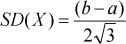# Uniform LawThe Uniform law is parameterized by a lower limit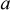(unit: millimeter) and a upper limit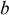(unit: millimeter). Another name for the Uniform law is Rectangular law.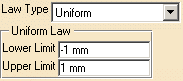Let's take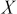a random variable following the Uniform law, then:is distributed according to the following density of probability, where: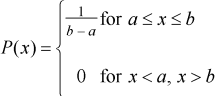With mean: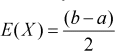With variance: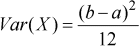With standard deviation: